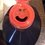# 0.99999999...=1 -- Infintesimal

This problem, while always leading to a wonderfully lively debate, does bring up the major problem of how you have valid proofs and still an idea that is fundamentally at odds with an intuitive understanding of how a created number system should work.

First off, it must be stated the proofs are (at least, as far as I can tell) unimpeachable--and any disputes stating the answer is wrong MUST also show how each of the proofs are fail to remain valid. I, by no means, am capable of achieving this; nor have I seen anyone (to date) that has posted such a rebuttal.

However, I would like to enter into evidence a concept referenced by an author of one of the formulations of this (current) identity (@Jake Lai)--the infinitesimal. This, for me, "solves" the problem; in fact, when pointed out, it is the perfect compliment to the infinite sum that underlies the more rigorous proofs.

The question, though, remains: What, exactly, does this mean for such an interpretation? Is not the infinitesimal used in the same way limits, imaginary numbers, and division by zero are?--i.e. as (temporary) work-arounds for shortcomings in the system as it stands?

This works, of course, as long as the destination actually DOES avoid (in the final accounting) the original paradox it was created to avoid. Of course, this isn't to say there aren't VALID interpretations where this is not the case--cf. Ramanujan Summation or Galois Theory--, but even here the applications require "interpretation" outside of the (current) number system. Would this application not also need to adhere to the same restriction of having either "net-error-avoidance", or at least "imprecise" application to the problem? [These are honest questions, as I am neither a mathematician, nor a student well-versed enough in number theory to seek verification on my own.]

So, in (forgive the pun) sum (hahah...no?...not even a little bit funny?):
1) Is the infinitesimal a valid "interpretation"?
2) [If 1 is true] How does the interpretation avoid the caveats above?
[Of course, the corollary question is--are the caveats above valid?]Note by Joshua Nesseth
4 years, 7 months ago

This discussion board is a place to discuss our Daily Challenges and the math and science related to those challenges. Explanations are more than just a solution — they should explain the steps and thinking strategies that you used to obtain the solution. Comments should further the discussion of math and science.

When posting on Brilliant:

• Use the emojis to react to an explanation, whether you're congratulating a job well done , or just really confused .
• Ask specific questions about the challenge or the steps in somebody's explanation. Well-posed questions can add a lot to the discussion, but posting "I don't understand!" doesn't help anyone.
• Try to contribute something new to the discussion, whether it is an extension, generalization or other idea related to the challenge.

MarkdownAppears as
*italics* or _italics_ italics
**bold** or __bold__ bold
- bulleted- list
• bulleted
• list
1. numbered2. list
1. numbered
2. list
Note: you must add a full line of space before and after lists for them to show up correctly
paragraph 1paragraph 2

paragraph 1

paragraph 2

[example link](https://brilliant.org)example link
> This is a quote
This is a quote
    # I indented these lines
# 4 spaces, and now they show
# up as a code block.

print "hello world"
# I indented these lines
# 4 spaces, and now they show
# up as a code block.

print "hello world"
MathAppears as
Remember to wrap math in $$ ... $$ or $ ... $ to ensure proper formatting.
2 \times 3 $2 \times 3$
2^{34} $2^{34}$
a_{i-1} $a_{i-1}$
\frac{2}{3} $\frac{2}{3}$
\sqrt{2} $\sqrt{2}$
\sum_{i=1}^3 $\sum_{i=1}^3$
\sin \theta $\sin \theta$
\boxed{123} $\boxed{123}$

Sort by:

@Jake Lai (See mention in the note)

Staff - 4 years, 7 months ago

First.- 0.999999... is not well- defined...

Second.- Every real number has one only decimal representation, for example, what is the decimal representation of 1?

a) 1.0

b) 1

c) 1.00

d) 1.000000000000000000000

Third.- it's usual on Brilliant, for instance, if you write 0.999999...., The ellipsis (suspension points) means that the number 9 is repeated an infinitum countable number of times after the last 9 written.

Fourth.- what is $\displaystyle \sum_{n = 1}^\infty \frac{9}{10^n} \space \text{?}$ 0.999999.....(This is a limit)? or 1?

P.S.- I hope this helps you. If someone wishes to reply me, he can do it, of course....

- 4 years, 6 months ago